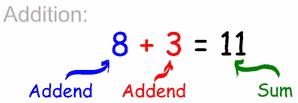# Basic Math — Addition

#### The Basic Operations

In basic mathematics there are many ways of saying the same thing:

Symbol
Words Used
+
Subtraction, Subtract, Minus, Less, Difference, Decrease, Take Away, Deduct
×
Multiplication, Multiply, Product, By, Times, Lots Of
÷
Division, Divide, Quotient, Goes Into, How Many Times

 The numbers to be added together are called the “Addends“